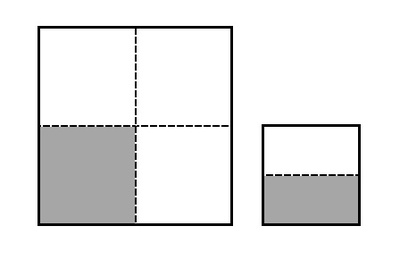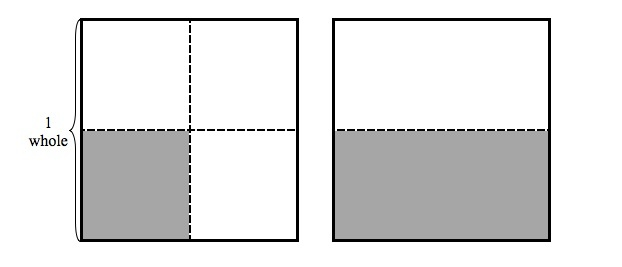Comparing Fractions with a Different Whole

Alignments to Content Standards: 3.NF.A.3.d

Bryce drew this picture:Then he said,

This shows that $\frac{1}{4}$ is greater than $\frac{1}{2}$.
1. What was his mistake? Draw a picture that shows why $\frac{1}{2}$ is greater than $\frac{1}{4}$.

2. Which of these comparison of $\frac{1}{4}$ with $\frac{1}{2}$ are true?
1. $\frac{1}{4} \gt \frac{1}{2}$
2. $\frac{1}{4} \lt \frac{1}{2}$
3. $\frac{1}{4} = \frac{1}{2}$
4. $\frac{1}{2} \gt \frac{1}{4}$
5. $\frac{1}{2} \lt \frac{1}{4}$

IM Commentary

This task is meant to address a common error that students make, namely, that they represent fractions with different wholes when they need to compare them. This task is meant to generate classroom discussion related to comparing fractions. Particularly important is that students understand that when you compare fractions, you implicitly always have the same whole. By recognizing the mistake that Bryce has made in his reasoning, students will demonstrate their understanding that comparisons between fractions are valid only when the fractions refer to the same whole. As they explain and correct the mistake in Bryce's reasoning, students will also demonstrate their ability to compare these unit fractions and record their comparisons using the correct symbols.

Solution

1. While it is true that the shaded region on the right has a smaller area than the shaded region on the left, the picture doesn't help us to compare $\frac{1}{4}$ and $\frac{1}{2}$. The reason is that the two wholes have different areas, and are thus different sizes.

In order to correctly compare these two fractions, we need to draw a picture of them with the same sized whole. The picture below correctly shows that $\frac{1}{2}$ is greater than $\frac{1}{4}$:2. The picture above shows the same whole divided into 4 pieces on the left and 2 pieces on the right. $\frac{1}{4}$ is shaded on the left and $\frac{1}{2}$ is shaded on the right. Because these two fractions refer to the same sized whole, it is easy to see that $\frac12$ is greater than $\frac14$. To be explicit about the reasoning, we know that $\frac{1}{4}$ and $\frac{1}{2}$ both represent 1 part but $\frac{1}{4}$ is 1 part when the whole is divided into 4 parts while $\frac{1}{2}$ is 1 part when the whole is divided into 2 parts. If a whole is divided into 4 equal parts, each part is smaller than if the same whole is divided into 2 parts. This means that 1 of 4 equal parts must be less than 1 of 2 equal parts of the same sized whole. Two of the given choices correctly illustrate this comparison using symbols. The correct choices are:

ii. $\frac14 \lt \frac12$ which says that $\frac14$ is less than $\frac12$, and

iv. $\frac12 \gt \frac14$which says that $\frac12$ is greater than $\frac14$.